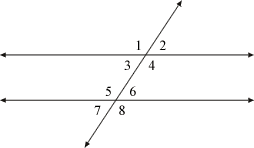index: click on a letter A B C D E F G H I J K L M N O P Q R S T U V W X Y Z A to Z index index: subject areas numbers & symbols sets, logic, proofs geometry algebra trigonometry advanced algebra & pre-calculus calculus advanced topics probability & statistics real world applications multimedia entrieswww.mathwords.com about mathwords website feedback

Consecutive Interior Angles

In the figure below, angles 4 and 6 are consecutive interior angles. So are angles 3 and 5. Consecutive interior angles are supplementary. Formally, consecutive interior angles may be defined as two interior angles lying on the same side of the transversal cutting across two parallel lines.

 Parallel lines cut by a transversal